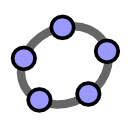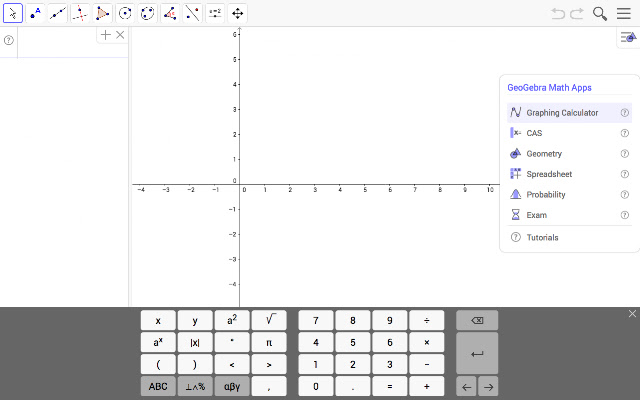Type of content Categories# GeoGebra Classic

More than 2684483 users worldwide
Current version: 6.0.640.0
Price: FREE
Like this platform_app ? Like/Share it!Apps bundle including free tools for geometry, spreadsheet, probability, and CASGeoGebra Classic joins graphing, geometry, 3D, spreadsheets, computer algebra and probability in one easy-to-use and powerful package. Millions of people around the world use GeoGebra to learn math and science. Join us!

GeoGebra Classic includes the following math tools:
* Graphing: plot functions with sliders and solve equations
* Geometry: create interactive geometric constructions
* 3D Graphing: graph functions, surfaces and many more 3D objects
* Spreadsheet: analyze data and do statistics connected with graphing
* CAS: solve math problems with our powerful computer algebra system
* Probability: visualize parameters and distributions quickly